Transaction Risk Capital in Commercial Lending

Barry Belkin, Larry R. Forest, Jr., Scott D. Aguais, and Stephan J. Suchower

In reference  we defined the unexpected total return (UTR) risk premium associated with a commercial loan. We showed that if the lender chooses to hold the loan but to transfer the associated unexpected return exposure to a counterparty, then the UTR risk premium for the loan can be identified as the cost of the required UTR swap under risk-neutral pricing.

In what follows we first show how one can impute an allocation of risk capital to a loan transaction from the UTR risk premium associated with the loan and the lender’s hurdle rate for excess return on capital. We then relate this imputed risk capital to that derived from the lender's internal risk capital rules and explore the properties of imputed risk capital through a series of simple examples. Finally, we provide two explicit portfolio management strategies that can be used to support loan origination decisions and loan hold/sell decisions.

Loan Transaction Risk Capital

Our point of departure is the following definition of the unexpected total return risk premium associated with a loan transaction: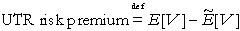(1) Here, E[V] denotes the expected net present value of the loan cash flows with respect to the natural probability measure for risk rating migration process of the borrower and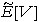denotes the counterpart expected net present value of the loan cash flows with respect to the risk-neutral measure for the borrower rating migration process. Since the unexpected total return risk premium is the only sense in which we use the term "risk premium," we will drop the "UTR" designation in what follows.

Suppose that the lender has set a fixed hurdle ratefor the return on capital he requires in excess of the risk-free rate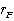. Let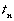(for) denote a sequence of valuation times such that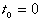(not necessarily the time of loan origination) and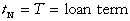. We then setLike the loan risk premium at time, the (transaction) risk capital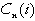allocated to the loan atdepends on the current risk grade of the borrower: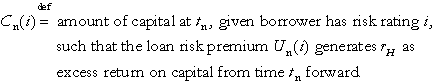Since rating migration is governed by a stochastic process, future values of the loan risk premium and of risk capital cannot be predicted with certainty. We therefore have to make precise the sense in which applying the risk premium to risk capital yields the return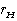in excess of the risk-free rate. The requirement we impose is that if the excess rate of return for capital is, then for any initial statethe expected present value of the aggregate dollar amount spent on risk capital from timeforward must be equal to the corresponding risk premium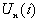.

This leads in a natural way to a recursive procedure for calculating the risk capital values. For this, we define: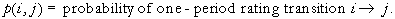The risk capital valuesare then related to the risk premiums by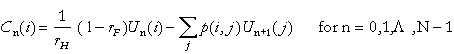(2)

(Note:.) Theare based on expected cash flow present values and can be efficiently determined using backward recursion methods.

As we have defined it, the risk capital allocated to a loan transaction is an imputed quantity determined in combination by (i) the risk premium that is available to support risk capital and (ii) the cost per unit time to "rent" risk capital. An immediate question is what relationship this imputed transaction risk capital bears to that obtained by applying the lender’s internal risk capital rules.

We make the assumption that the lender’s par credit spreads (both spot and forward) are calibrated to his internal risk capital rules as applied to standardized (option-free) term loans of varying maturity and credit quality. For these standardized loans, the imputed risk capital and that specified by the lender’s risk capital rules are the same.

The difficulty arises in applying risk capital rules to loans with structural complexities, such as the option for the borrower to prepay, grid pricing, and loan covenants. It is likely that the lender’s risk capital rules lack the resolution to capture such fine elements of loan structure. We argue that a reasonable alternative to applying rules that are insensitive to key elements of loan structure is to impute risk capital in the manner we have described. The rationale is twofold. First, the risk premium on which imputed risk capital is based fully reflects loan structure. Second, the use of risk-neutral pricing in imputing risk capital is consistent with the absence of internal arbitrage opportunities within the lender’s loan portfolio. We thus take the point of view that our method for imputing risk capital to loan transactions is an appropriate way to expand the "repertoire" of the lender’s risk capital rules.

To this point we have focused on the par credit spreads a lender derives from his internal risk capital rules. These internal par credit spreads are likely to differ, perhaps substantially, from those observed in the loan market. A question then is what risk capital rules is the market applying to justify its par credit spreads. The market, of course, does not directly reveal its risk capital rules. However, a set of such rules is implicit in the market par credit spreads and market (excess) return on risk capital. (We note that the long-term average return on capital for the well-diversified "market portfolio" of corporate stocks as cited in reference  is 8.4% over the risk-free rate.)

To get at these imputed market risk capital rules, consider a hypothetical lender who holds a well-diversified loan portfolio approximating the "market portfolio," who sets his par credit spreads equal to those of the market, and who sets his hurdle rate for excess return on risk capital equal to the market excess return on capital. If one applies the methods we have described for imputing risk capital to the lender’s portfolio, one obtains the imputed market risk capital rules.

In general, a lender should expect to allocate more capital to a loan than the imputed market capital (and pay more for that capital than the market rate). By comparing the amount of risk capital he is required to allocate to a given loan under his internal risk capital rules with the amount of risk capital the market would impute to the same loan, a lender can gain some insight into the penalty he pays for not achieving the same level of portfolio diversification as the market.

Following  we take the view that the lender maintains two separate internal "accounts": an operating account to manage loan cash flows and a capital account to support the risk inherent in those cash flows. The operating account can be viewed as paying the risk premium to the capital account to enter into an internal unexpected total return swap as previously described. This swap transfers all of the risk associated with the loan cash flows to the capital account.

We carry the analysis a step further by merging the financial "statements" of the two accounts in order to determine the consolidated return on risk capital for the loan. It is this consolidated return that we refer to as the risk-adjusted return on capital or RAROC value (denoted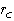) for the loan.

The various definitions we have introduced imply the relationships summarized in Table 1 below.

Table 1: Relationship Between NPV and RAROC Views of Loan Value

 Expected Cash Flow Into Capital Account Excess Return on Risk Capital Expected Residual Value in Operating Account Risk Premium: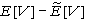Hurdle Rate:Loan Market Value:Consolidated Cash Flow Value: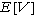RAROC:0

The most important of the relationships in Table 1 is that between the NPV view and the RAROC view of loan value:(3)

Equation (3) states that a par loan, i.e., a loan that has zero expected net present value under market (risk-neutral) valuation, is equivalently a loan that yields the lender’s hurdle rate as its RAROC value. This parity between the NPV and RAROC views of loan value is a consequence of our assumption that a par loan must yield the lender’s hurdle rate as return on risk capital.

Numerical Examples

We now turn to some simple numerical examples to investigate the effect of loan price and structure on risk capital and RAROC. We restrict attention to an option-free two-year term loan with \$10,000 principal and annual cash flows. We assume a seven passing grade credit rating system and par credit spreads, a deterministic value of 40% for loss in the event of default (LIED), and a constant risk-free rate of 4.5%. We neglect loan origination/monitoring costs and operating expense. In addition, we take the lender hurdle rate for excess return on capital to be 15%.

In Table 2 below we show how risk capital is affected by the remaining loan term. We consider three variations: (i) the (fixed) risk capital for a one-year loan, (ii) the first year risk capital for a two-year loan, and (iii) the expected second year risk capital for a two-year loan.

Table 2: Effect of Remaining Loan Term on Transaction Risk Capital

Borrower Risk Rating at Loan Origination
 Aaa Aa A Baa Ba B Caa One-Year Loan: Risk Capital \$.68 \$2.00 \$7.33 \$14.67 \$66.67 \$300.00 \$733.33 Two-Year Loan: Year 1 Risk Capital \$.68 \$2.00 \$7.35 \$14.74 \$67.04 \$301.89 \$741.01 Two-Year Loan: Expected Year 2 Risk Capital \$.97 \$2.67 \$8.44 \$19.45 \$78.91 \$285.99 \$593.22

The first observation based on Table 2 is that for each borrower risk rating at loan origination, the risk capital for year 1 of the two-year loan is equal to or marginally greater than the risk capital for the one-year loan. In the first year, both loans are exposed to the same risk of loss of principal if default occurs. However, if a first-year default occurs on a two-year loan, there is the added exposure to the loss (in present value) of the net loan revenue that would otherwise have accrued in the second year.

We next observe that in the case of the two-year loan, there is a significant difference between the first year risk capital and the expected second year risk capital. For initial rating grades Aaa through Ba, the expected second year risk capital is higher. For grades B and Caa, the relationship is reversed, and the first year risk capital is higher. For grades Aaa through Ba, the dominant effect is that of possible downward migration (short of default) in year 1, with a resulting increased exposure in year 2. For grades B and Caa, the dominant effect is that of upward migration (reducing the exposure in year 2) or default (eliminating any exposure in year 2).

The final point to be made based on Table 2 is that variation in risk capital with borrower rating is far more significant than the variation in risk capital with the remaining term of the loan. One observes that risk capital increases by a factor of about 1,000 in going from a Aaa borrower down to a Caaborrower, reflecting the much higher probability of default.

In Table 3 we examine how the risk premium, initial risk capital, and RAROC for a loan are affected by pricing. The borrower rating at loan origination is fixed at Caa (the rating at which the effect of pricing is most pronounced) and the loan spread is varied in 10 basis point increments about the par spread of 632 bp.

Table 3: Effect of Loan Spread on Risk Premium, Risk Capital, and RAROC

Loan principal: \$10,000

Loan term: 2 years

Initial borrower credit rating: Caa

 Loan Spread (bps) Risk Premium Initial Risk Capital RAROC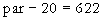\$187.35 \$737.52 12.24%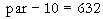\$187.60 \$739.26 13.62%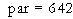\$187.85 \$741.01 15.00%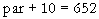\$188.10 \$742.76 16.37%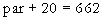\$188.35 \$744.50 17.74%

One observes from Table 3 that the loan risk premium, initial risk capital, and RAROC are each increasing functions of the loan spread. In each case, the variation is approximately linear in the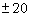bp neighborhood of the par spread shown. Both the risk premium and the initial risk capital are seen to be relatively insensitive to changes in the loan pricing. A 10 bp change in spread induces a \$.25 change in risk premium and a \$1.75 change in risk capital.

By contrast, the dependence of RAROC is pronounced — about 1.38% increase in RAROC per 10 bp increase in the loan spread. The overall implication of Table 3 is that very little of a loan price increase is lost to the associated increase in the cost of risk capital. Correspondingly, very little of a price decrease is recovered by the lender through the associated reduction in the cost of risk capital.

So far, we have focused our attention entirely on the individual loan transaction. To gain further insight into the workings of risk capital, it is instructive to consider the implications of our definition of risk capital at the portfolio level.

For this purpose, we imagine two banks (A and B), such that bank A maintains a portfolio of exclusively one-year term loans, while B maintains a portfolio of exclusively two-year term loans. Both banks follow the policy of originating loans only to borrowers with a specific rating grade. Borrowers who migrate out of the specific rating class (including those who default) do not have their loans renewed. Rather, they are replaced by new borrowers with the required rating grade.

Suppose, for example, that banks A and B each decide to originate loans only to borrowers with risk rating Ba. Under our assumptions, at any point in time, bank A will hold a portfolio made up exclusively of Ba loans. Bank B, on the other hand, will hold a mixture, in roughly equal proportions, of two-year loans in their first year (all Ba rated) and two-year loans in their second year (mostly Ba rated).

The question we address in Table 4 is how the risk capital requirements of the two bank compare. Results are shown for each possible origination rating grade.

Table 4: Effect of Loan Term on Portfolio Risk Capital

Value shown is average risk capital per \$10,000 loan

Borrower Risk Rating at Loan Origination
 Aaa Aa A Baa Ba B Caa Bank A Portfolio: One-Year Loans \$.67 \$2.00 \$7.33 \$14.67 \$66.67 \$300.00 \$733.33 Bank B Portfolio: Two-Year Loans \$.83 \$2.34 \$7.89 \$17.09 \$72.93 \$294.10 \$670.72

Interestingly, the results in Table 4 show that which of the two banks would hold more risk capital depends on the rating class of borrowers to which they originate loans. If that rating class is in the range Aaa to Ba, then bank B would require the greater risk capital. If the two banks decide to go down market and originate loans only to B or only to Caa borrowers, then bank A would have to hold the greater risk capital.

In the more realistic case where both banks originated loans to borrowers of all rating classes but maintained their strict policies with regard to loan term, which one of the two banks requires more risk capital would depend on the mix of borrower ratings in the portfolios of the two banks.

The point to be made from Table 4 is that the decision by bank B to write only two-year loans does not automatically imply that bank B must hold more risk capital than bank A, which writes only one-year loans. This behavior is consistent with our open-end loan portfolio paradigm, under which the lender renews maturing loans and originates new loans to maintain an effective portfolio composition equilibrium. Under the open-end portfolio paradigm, risk capital is driven by instantaneous risk exposure.

This contrasts sharply with the closed-end portfolio paradigm, where the lender is presumed to hold a fixed set of loans until they mature. If bank A and bank B were operating under the closed-end paradigm and were seeking to liquidate their respective portfolios, then it certainly would be the case that bank B would have the greater cumulative credit risk exposure and would be forced to hold the larger amount of risk capital.

Risk Capital at the Portfolio Level

The important question remains whether the aggregate portfolio risk capital that results from these individual capital allocations at the transaction level is actually adequate to cover the lender’s exposure to credit risk.

There are various ways for a lender to judge the adequacy of his portfolio risk capital. One approach is for the lender to set a target value for his rating agency credit grade and then determine whether he holds sufficient risk capital to justify that credit grade. A specific risk horizon is chosen (one year is typical). Relative to this risk horizon, let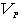denote the value of the lender’s loan portfolio,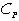denote the risk capital the lender allocates to his loan portfolio, and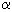denote the published probability of default for the target risk rating.

Let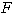be the probability distribution for. Then the test for the adequacy of the lender’s portfolio risk capital is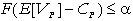,                   (4) i.e., the probability must be at most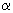that the lender’s portfolio risk capital will be insufficient to cover any shortfall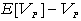in the value of his loan portfolio relative to expectation. We denote the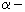quantile ofby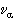. Following general practice (see reference ), we refer toas the portfolio value at risk (VAR).

One way to quantify the credit exposure attributable to an individual transaction is to determine its effect on VAR. For this we define the following quantities (all relative to the risk horizon):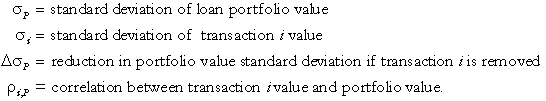Then the following relationship holds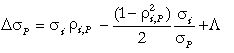.              (5) If the relative contribution of any single transaction to the volatility of the overall portfolio is small, then to a close approximation(6)

Suppose that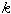has been chosen so that the amount of portfolio risk capitalproduces exact equality in (4) and just earns the lender his targeted credit rating grade. Then based on (6), the appropriate amount of risk capital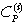for the lender to allocate to transaction i is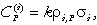(7) (Note: Since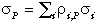, equation (7) defines a partitioning of portfolio risk capital into amounts of transaction risk capital.)

We previously described how to impute an amount of risk capital to a transaction consistent with the lender’s targeted hurdle rate. Equation (7) provides an alternative way to assign risk capital to a transaction based on its relative contribution to the portfolio exposure. We refer to the risk capital as given by equation (7) as the exposure risk capital allocated to the transaction.

Our analysis here in combination with that in reference  supports two separate tests to apply to a transaction:

value test: originate (or buy) a transaction if it has a positive expected net present value under the risk-neutral measure calibrated to the market par credit spreads.

exposure test: hold transaction in portfolio if its exposure risk capital is less than or equal to its imputed risk capital.

Note that in applying the value test, it is the market par credit spreads that are relevant since value is measured in relation to market pricing. A "good" loan to originate is one that the lender should be able sell at a profit. In applying the exposure test, it is the lender’s internal par credit spreads that are relevant. A "good" deal to hold is one that is "risk efficient" in the sense that the actual exposure it adds to the lender’s portfolio is at most that implied by the lender’s internal pricing of risk. Since imputed risk capital and exposure risk capital are equal for the standardized loans used to calibrate the lender’s internal par credit spreads, the standardized loans will necessarily pass the exposure test.

We observe that a transaction can pass the value test but fail the exposure test. The incentive then is for the lender to originate (or buy) the transaction but then sell it for a quick profit. The lender does not want to hold the deal in his portfolio because he does not expect to be adequately compensated for the incremental exposure it adds to his portfolio.

It is also the case that certain transactions in the lender’s portfolio can pass the exposure test but fail the value test. For such a deal, the expected loss is a sunk cost. The lender can realize the loss by selling the deal immediately or defer the loss by holding onto the loan. If the lender chooses to hold the deal, he will at least be adequately compensated for the incremental risk capital it ties up.

It is certainly possible that many (perhaps most) of the transactions in a given lender’s portfolio will fail the exposure test. That would suggest an inconsistency between the return on capital required by the lender’s shareholders and the "risk efficiency" of the portfolio. Given the risk characteristics of the lender’s portfolio, the lender’s shareholders may be unwilling to accept a lower return on risk capital than the target hurdle rate. The lender would then be obliged to eliminate from his portfolio those loans that are the most "risk inefficient" in an effort to improve the portfolio return on capital.

Possible Extensions

There are a number of directions in which our approach to measuring credit risk can be extended.

We have assumed that LIED is deterministic. The calculation of the risk-neutral measure for credit rating migration then follows reference . However, we have been able to extend the methods of  to the case in which LIED is a random variable with known mean and variance. The definitions of risk premium, risk capital, and RAROC then directly carry over.

The risk-free rate used to discount cash flows has been assumed fixed. It is possible to combine an arbitrage-free stochastic interest rate model (such as the Heath-Jarrow-Morton model in reference ) with the arbitrage-free credit migration model we have described to obtain a joint model for interest rate risk and credit risk. Again, our basic definitions carry over without change. However, with more state variables required to describe the stochastic evolution of loan value, the joint model entails a significant increase in computation.

Our focus has been primarily on commercial loans. However, the framework we have described for measuring credit risk extends to bonds, credit derivatives, and generally to any structured set of cash flows subject to credit risk. The key requirement is that one must be able to calculate the expected net present value of those cash flows under both the natural and risk-neutral rating migration process measures in order to obtain the associated risk premium.

The authors are currently working on a factor model for credit rating migration that builds on the continuous state model for risk rating described in reference . The model incorporates a single "business cycle" factor that drives the stochastic evolution of rating grade transition probabilities, LIED distribution parameters, and par credit spreads. It also accounts for the correlation between the credit migration processes of different borrowers without imposing the requirement that the probability distribution for portfolio value at the risk horizon be Gaussian.

Summary

We have introduced specific definitions for three important quantities related to the measurement of credit risk at the transaction level: risk premium, risk capital, and risk-adjusted return on capital (RAROC). The key element in our approach is linking (through risk-neutral pricing methods) the marginal pricing of credit risk implicit in one-period par credit spreads to the pricing of the aggregate risk inherent in a multi-period credit instrument.

We have identified the risk premium associated with a credit transaction as the cost of a form of total return swap which transfers the unexpected return risk on the trans-action to a counterparty (which could be the lender’s risk capital account). This risk premium supports an imputed amount of risk capital consistent with the lender realizing a targeted hurdle rate as excess return on capital. We differentiated this imputed transaction risk capital from the exposure risk capital allocated to the transaction based on consideration of the risk characteristics of the lender’s credit portfolio and his targeted credit rating. We defined the RAROC associated with a loan as the excess return on imputed risk capital generated by the loan revenue. We then explored the properties and interrelationships of these risk quantities through a series of simple examples based on a two-year term loan.

Two loan portfolio management guidelines emerged from our analysis: (1) originate (or buy) loans with positive expected net present value under the risk-neutral credit migration process measure (i.e., loans for which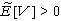) and (2) hold those loans for which exposure risk capital is less than or equal to imputed risk capital.

References

 Belkin, B., L. R. Forest, Jr., S. D. Aguais, and S. J. Suchower, "Credit Risk Premiums in Commercial Lending," Risk, to appear.

 Modigliani, F. and M. H. Miller, "The Cost of Capital, Corporation Finance and the Theory of Investment," American Economic Review, 48, June 1958, pp. 261-297.

 Brealey, R. A. and S. C Myers, "Principles of Corporate Finance." McGraw Hill, 1996

 KPMG Peat Marwick, "VAR: Understanding and Applying Value-at-Risk," Risk Publications, 1997.

 Belkin, B., S. J. Suchower, and L. R. Forest, Jr. "The Effect of Systematic Credit Risk on Loan Portfolio Value-at-Risk and Loan Pricing." CreditMetrics® Monitor, First Quarter 1998, pp.17-28

 Ginzburg, A., K. J. Maloney, and R. Willner. "Debt Rating Migration and the Valuation of Commercial Loans." Citibank Portfolio Strategies Group Report, December 1994

 Heath, D., R. Jarrow, and A. Morton, "Bond Pricing and the Term Structure of Interest Rates: A New Methodology for Contingent Claims Valuation," Econometrics, Vol. 60, No. 1, January 1992, pp. 77-105.

 J. P. Morgan & Co. Incorporated, "CreditMetricsTM: The Benchmark for Understanding Credit Risk," 1997.